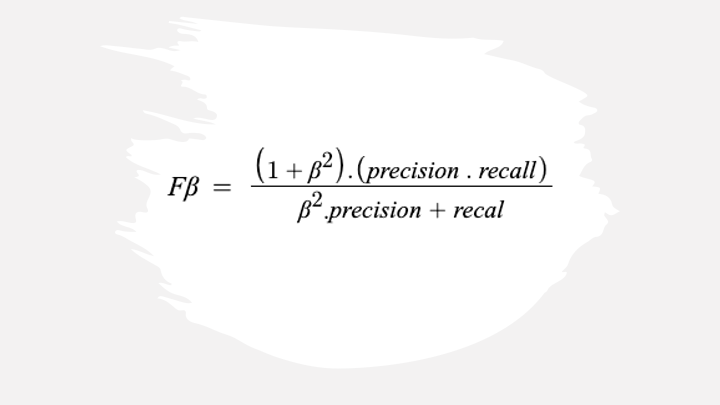Join thousands of AI enthusiasts and experts at the Learn AI Community.

## PublicationLatest

# F1 to F-beta

Last Updated on October 10, 2022 by Editorial Team

#### Author(s): Saurabh Saxena

Originally published on Towards AI the World’s Leading AI and Technology News and Media Company. If you are building an AI-related product or service, we invite you to consider becoming an AI sponsor. At Towards AI, we help scale AI and technology startups. Let us help you unleash your technology to the masses.

### F1 Score

The F-1 score is a popular binary classification metric representing a balance between precision and recall. It is the Harmonic mean of precision and recall. The following equation can represent the F-1 score.

where Precision can be defined as the probability of positive predictions that are actual members of the positive class.

The recall is defined as the probability of the positive predictions among the actual positive.

where TP is True Positive, FP is False Positive, and FN is the False Negative.

Let’s explore the F1 score for the binary classification problems with a dummy dataset in sklearn.

`from sklearn.datasets import make_classificationfrom sklearn.model_selection import train_test_splitfrom sklearn.linear_model import LogisticRegressionfrom sklearn.metrics import f1_score`
`X, y = make_classification(n_samples=1000, n_classes=2,                           random_state=1)X_train, X_test, y_train, y_test = train_test_split(X, y,                                                    test_size=.2,                                                    random_state=2)lr = LogisticRegression()lr.fit(X_train, y_train)y_pred = lr.predict(X_test)y_pred_prob = lr.predict_proba(X_test)y_pred_prob = y_pred_prob[:,1]f1_score(y_test, y_pred)`
`Output:0.8585858585858585`

While many Machine Learning and Deep Learning practitioners frequently use the F1 score for model evaluation, few are familiar with the F-measure, which is the general form of the F1 Score.

F-beta Score

The F-beta score calculation follows the same form as the F1 score. Unlike in F1 Score, which is the harmonic mean, it is the weighted harmonic mean of the precision and recall, reaching its optimal value at 1 and worst value at 0.

The beta parameter determines the weight of recall in the combined score. beta < 1 lends more weight to precision while beta > 1 favors recall.

Let’s have a look at the F-beta score and how the value fluctuates with beta.

`from sklearn.metrics import fbeta_score`
`print(fbeta_score(y_test, y_pred, beta=0.5))print(fbeta_score(y_test, y_pred, beta=1))print(fbeta_score(y_test, y_pred, beta=2))`
`Output:0.8534136546184740.85858585858585850.8638211382113821`

Here, we have noticed that F-beta changes with beta movement, and now let’s have a look at the same relative to precision and recall curve at various thresholds.

`import matplotlib.pyplot as pltfrom sklearn.metrics import recall_scorefrom sklearn.metrics import precision_scorefrom sklearn.metrics import precision_recall_curve`
`_, _, threshold = precision_recall_curve(y_test, y_pred_prob)`
`f1score = list()f05score = list()f2score = list()precision = list()recall = list()for th in threshold:                                                        y_test_pred = list()    for prob in y_pred_prob:        if prob > th:            y_test_pred.append(1)        else:            y_test_pred.append(0)        f1score.append(f1_score(y_test, y_test_pred))    precision.append(precision_score(y_test, y_test_pred))    recall.append(recall_score(y_test, y_test_pred))    f05score.append(fbeta_score(y_test, y_test_pred, beta=0.5))    f2score.append(fbeta_score(y_test, y_test_pred, beta=2))`
`_, ax = plt.subplots(figsize=(8, 6))ax.set_xlabel('Threshold')plt.plot(threshold, precision, label='precision')plt.plot(threshold, recall, label='recall')plt.plot(threshold, f05score, label='F0.5')plt.plot(threshold, f1score, label='F1')plt.plot(threshold, f2score, label='F2')plt.legend(loc='lower left')`

It is evident in the above graph that as we increase our beta value from 0, the curve starts moving towards the recall curve, which means with an increase in the beta value gives more importance to recall, and the below code to plot the F-measure at various beta and threshold values.

`betas = [0.1, 0.3, 0.5, 0.7, 1, 2, 5]_, ax = plt.subplots(figsize=(8, 6))ax.set_xlabel('Threshold')ax.set_ylabel('Fbeta')for beta in betas:    fbetascore = list()    for i, th in enumerate(threshold):        y_test_pred = list()        for prob in y_pred_prob:            if prob > th:                y_test_pred.append(1)            else:                y_test_pred.append(0)        fbetascore.append(fbeta_score(y_test, y_test_pred,                                      beta=beta))    plt.plot(threshold, fbetascore, label=f'F{beta}')plt.legend(loc='lower left')`

References:F1 to F-beta was originally published in Towards AI on Medium, where people are continuing the conversation by highlighting and responding to this story.

Join thousands of data leaders on the AI newsletter. It’s free, we don’t spam, and we never share your email address. Keep up to date with the latest work in AI. From research to projects and ideas. If you are building an AI startup, an AI-related product, or a service, we invite you to consider becoming a sponsor.

Published via Towards AI